# 3.4 Perturbation Expansion of the GREEN's Function

In previous sections GREEN's functions at zero and finite temperatures have been defined. It was shown that the GREEN's functions can be written in terms of the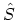operator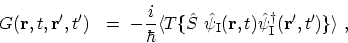(3.28)

whereincludes the effects of interactions and external perturbations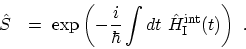(3.29)

Unfortunately, it is not possible to give an analytical solution for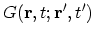, unless the interaction perturbation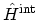is set equal to zero. This gives the non-interacting GREEN's function (see Appendix D)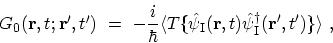(3.30)

which is central for any perturbation expansion.

This section proceeds the calculation of the GREEN's function by expanding theoperator as series of products of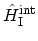in the numerator and the denominator. By expanding theoperator one obtains (see (B.20))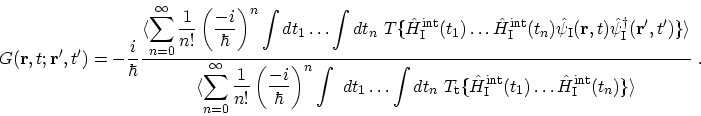(3.31)

The expansion of the numerator of the GREEN's function in (3.31) can be written as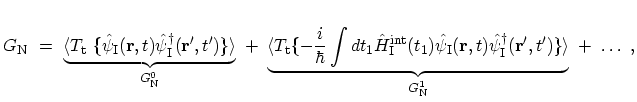(3.32)

where the superscript denotes the order of perturbation. The zero-order perturbation leads to non-interacting GREEN's function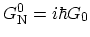. WICK's theorem allows us to write each of these brackets in terms of non-interacting GREEN's function and the interaction potential. The same procedure can be applied to the denominator. The terms in the expansion of the denominator,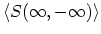, are called vacuum polarization terms .

Different expansion terms achieved from the WICK theorem can be translated into FEYNMAN diagrams (Appendix E). FEYNMAN introduced the idea of representing different contributions obtained from the WICK decomposition by drawings. These drawings, called diagrams, are very useful for providing an insight into the physical processes which these terms represent. The FEYNMAN diagrams provide an illustrative way to solve many-body problems and the perturbation expansion of GREEN's functions.

Subsections

M. Pourfath: Numerical Study of Quantum Transport in Carbon Nanotube-Based Transistors